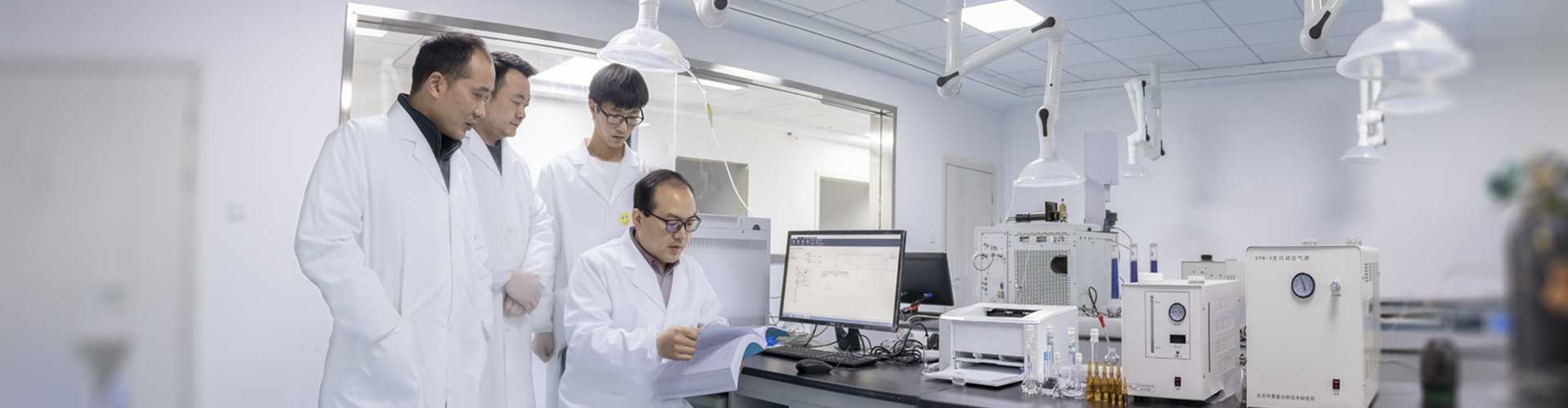• 首页

bzwz.com
• 产品与服务

100,000+
• 新闻

news
• 交流区

community
• 课堂

Course
• 会员中心

account
• 关于我们##等度洗脱和梯度洗脱的选择及条件计算

020分钟内，将有机相比例从5-10%线性升高至100%，并保持一段时间以保证所有组分被洗脱出来。1、等度洗脱如何设置tr(avg)=(ti+tf)/2=17min

Δ%B/min=(%Bfinal-%Binitial)/tG=(100-5)/20=4.75 %B/min

2梯度洗脱如何设置Δ%B/min=(%Bfinal-%Binitial)/tG=(100-5)/20=4.75 %B/min

Optimized%Binitial

=(%Binitial+(tixΔ%B/min))-(V/FxΔ%B/min)-10%B

=(5+(12.786x4.75))-(5.5/2x4.75)-10

=42.67%B

Optimized%Bfinal

=(%Binitial+(tfxΔ%B/min))-(V/FxΔ%B/min)-10%B

=(5+(21.142x4.75))-(5.5/2x4.75)-10

=82.36%B

0 收藏 回复：0 阅读：288
• 暂无回复...

• ### 外来入侵植物对自然保护区植物多样性影响评估技术导则（征求意见稿）

1.手机直接输入，座机前请加区号 如13803766220,010-58103678

2.我们将根据您提供的电话号码，立即回电，请注意接听

3.因为您是被叫方，通话对您免费，请放心接听